# Fraction calculator

The calculator performs basic and advanced operations with fractions, expressions with fractions combined with integers, decimals, and mixed numbers. It also shows detailed step-by-step information about the fraction calculation procedure. Solve problems with two, three, or more fractions and numbers in one expression.

## Result:

### 1/8 - 1/12 = 1/24 ≅ 0.04166667

Spelled result in words is one twenty-fourth.

### How do you solve fractions step by step?

1. Subtract: 1/8 - 1/12 = 1 · 3/8 · 3 - 1 · 2/12 · 2 = 3/24 - 2/24 = 3 - 2/24 = 1/24
For adding, subtracting, and comparing fractions, it is suitable to adjust both fractions to a common (equal, identical) denominator. The common denominator you can calculate as the least common multiple of both denominators - LCM(8, 12) = 24. In practice, it is enough to find the common denominator (not necessarily the lowest) by multiplying the denominators: 8 × 12 = 96. In the following intermediate step, it cannot further simplify the fraction result by canceling.
In other words - one eighth minus one twelfth = one twenty-fourth.

#### Rules for expressions with fractions:

Fractions - simply use a forward slash between the numerator and denominator, i.e., for five-hundredths, enter 5/100. If you are using mixed numbers, be sure to leave a single space between the whole and fraction part.
The slash separates the numerator (number above a fraction line) and denominator (number below).

Mixed numerals (mixed fractions or mixed numbers) write as integer separated by one space and fraction i.e., 1 2/3 (having the same sign). An example of a negative mixed fraction: -5 1/2.
Because slash is both signs for fraction line and division, we recommended use colon (:) as the operator of division fractions i.e., 1/2 : 3.

Decimals (decimal numbers) enter with a decimal point . and they are automatically converted to fractions - i.e. 1.45.

The colon : and slash / is the symbol of division. Can be used to divide mixed numbers 1 2/3 : 4 3/8 or can be used for write complex fractions i.e. 1/2 : 1/3.
An asterisk * or × is the symbol for multiplication.
Plus + is addition, minus sign - is subtraction and ()[] is mathematical parentheses.
The exponentiation/power symbol is ^ - for example: (7/8-4/5)^2 = (7/8-4/5)2

#### Examples:

subtracting fractions: 2/3 - 1/2
multiplying fractions: 7/8 * 3/9
dividing Fractions: 1/2 : 3/4
exponentiation of fraction: 3/5^3
fractional exponents: 16 ^ 1/2
adding fractions and mixed numbers: 8/5 + 6 2/7
dividing integer and fraction: 5 ÷ 1/2
complex fractions: 5/8 : 2 2/3
decimal to fraction: 0.625
Fraction to Decimal: 1/4
Fraction to Percent: 1/8 %
comparing fractions: 1/4 2/3
multiplying a fraction by a whole number: 6 * 3/4
square root of a fraction: sqrt(1/16)
reducing or simplifying the fraction (simplification) - dividing the numerator and denominator of a fraction by the same non-zero number - equivalent fraction: 4/22
expression with brackets: 1/3 * (1/2 - 3 3/8)
compound fraction: 3/4 of 5/7
fractions multiple: 2/3 of 3/5
divide to find the quotient: 3/5 ÷ 2/3

The calculator follows well-known rules for order of operations. The most common mnemonics for remembering this order of operations are:
PEMDAS - Parentheses, Exponents, Multiplication, Division, Addition, Subtraction.
BEDMAS - Brackets, Exponents, Division, Multiplication, Addition, Subtraction
BODMAS - Brackets, Of or Order, Division, Multiplication, Addition, Subtraction.
GEMDAS - Grouping Symbols - brackets (){}, Exponents, Multiplication, Division, Addition, Subtraction.
Be careful, always do multiplication and division before addition and subtraction. Some operators (+ and -) and (* and /) has the same priority and then must evaluate from left to right.

## Fractions in word problems:

• Sewing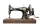The lady cut off one-half of the cloth. She needed three-quarters of this piece to sew a skirt. What part of the original piece of cloth remained?
• EquationSolve equation and check the result: 1.4x - 3/2 + x - 9,8 = x + 0,4/3 - 7 + 1,6/6
• The bunsKate, Zofia, and Peter Liked buns. Even today, their grandmother prepares their favorite meal. Katka eats 4 bunches, Žofia 3, and Petra eats 5 buns. Their grandmother said to them, "My inmate will you know how many buns I have been made today if those you
• Class 8.AThree quarters of class 8.A went skiing. Of those who remained at home one third was ill and the remaining six were on math olympic. How many students have class 8.A?
• Honza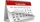Honza is 13 years old and Peter 21 years old. After how many years are their ages will be at ratio 7: 9?
• Discount saleAfter the discount, the computer costs 9600, - CZK. How much did it cost when the price was reduced: a) by half b) by a third c) by one fifth and then by 160 CZK
• Division of moneyCalculate how many euros have Matthew, Miriam, Lucy, Michael, Janka when together have 2,700 euros and the amounts are at a ratio of 1:5:6:7:8.
• Three men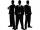Alex is half younger than Jan, which is one-third younger than George. The sum of their ages is 48. How are these three men old?
• Sixty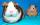Sixty percent of one-fifteen of the total is equal to thirty. What are two percent of the total?
• Cyclist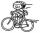The cyclist goes uphill 10 km for 50 minutes and downhill minutes for 29 minutes, both applied to the pedals the same force. How long he pass 10 km by plane?
• MO Z9–I–2 - 2017In the VODY trapezoid, VO is a longer base and the diagonal intersection K divides the VD line in a 3:2 ratio. The area of the KOV triangle is 13.5 cm2. Find the area of the entire trapezoid.
• Evaluate expressionCalculate the value of the expression z/3 - 2 z/9 + 1/6, for z = 2
• Denominator 2Denominator of a fraction is 5 and numerator is 7. Write the fraction .